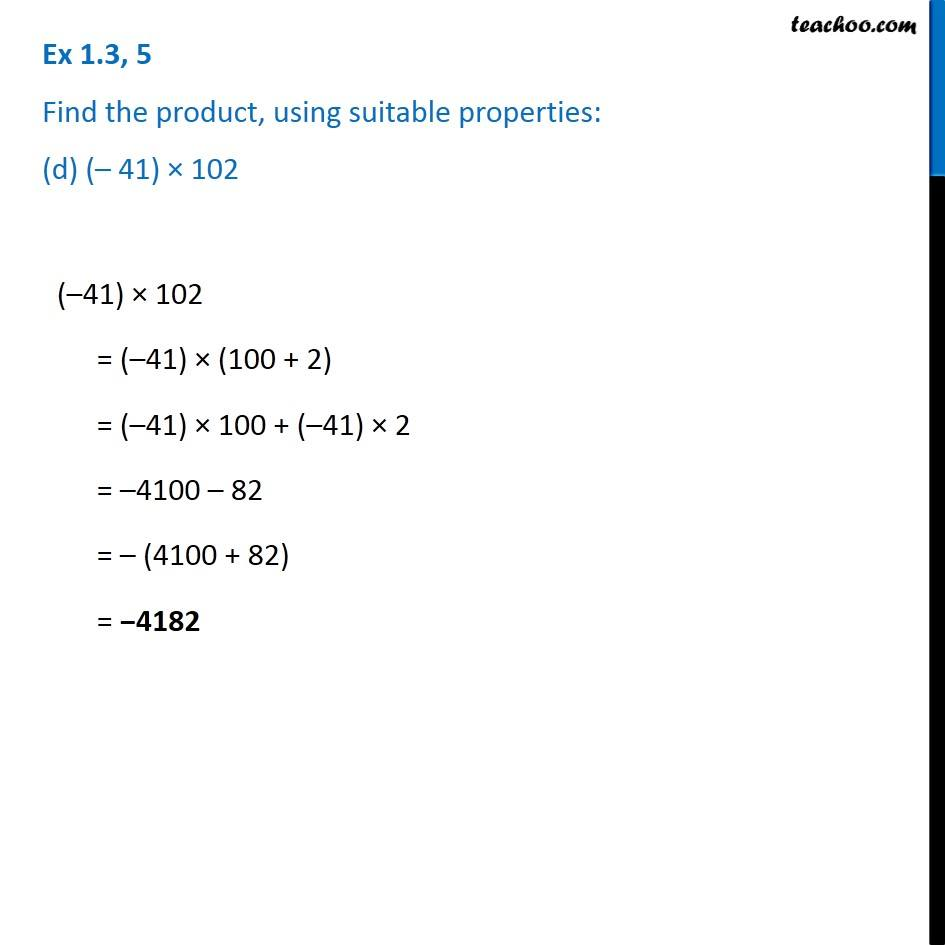1. Chapter 1 Class 7 Integers
2. Serial order wise
3. Ex 1.3

Transcript

Ex 1.3, 5 Find the product, using suitable properties: (d) (– 41) × 102 (–41) × 102 = (–41) × (100 + 2) = (–41) × 100 + (–41) × 2 = –4100 – 82 = – (4100 + 82) = −4182